$$\newcommand{\id}{\mathrm{id}}$$ $$\newcommand{\Span}{\mathrm{span}}$$ $$\newcommand{\kernel}{\mathrm{null}\,}$$ $$\newcommand{\range}{\mathrm{range}\,}$$ $$\newcommand{\RealPart}{\mathrm{Re}}$$ $$\newcommand{\ImaginaryPart}{\mathrm{Im}}$$ $$\newcommand{\Argument}{\mathrm{Arg}}$$ $$\newcommand{\norm}{\| #1 \|}$$ $$\newcommand{\inner}{\langle #1, #2 \rangle}$$ $$\newcommand{\Span}{\mathrm{span}}$$

# 1.1E: Exercises

$$\newcommand{\vecs}{\overset { \rightharpoonup} {\mathbf{#1}} }$$ $$\newcommand{\vecd}{\overset{-\!-\!\rightharpoonup}{\vphantom{a}\smash {#1}}}$$$$\newcommand{\id}{\mathrm{id}}$$ $$\newcommand{\Span}{\mathrm{span}}$$ $$\newcommand{\kernel}{\mathrm{null}\,}$$ $$\newcommand{\range}{\mathrm{range}\,}$$ $$\newcommand{\RealPart}{\mathrm{Re}}$$ $$\newcommand{\ImaginaryPart}{\mathrm{Im}}$$ $$\newcommand{\Argument}{\mathrm{Arg}}$$ $$\newcommand{\norm}{\| #1 \|}$$ $$\newcommand{\inner}{\langle #1, #2 \rangle}$$ $$\newcommand{\Span}{\mathrm{span}}$$ $$\newcommand{\id}{\mathrm{id}}$$ $$\newcommand{\Span}{\mathrm{span}}$$ $$\newcommand{\kernel}{\mathrm{null}\,}$$ $$\newcommand{\range}{\mathrm{range}\,}$$ $$\newcommand{\RealPart}{\mathrm{Re}}$$ $$\newcommand{\ImaginaryPart}{\mathrm{Im}}$$ $$\newcommand{\Argument}{\mathrm{Arg}}$$ $$\newcommand{\norm}{\| #1 \|}$$ $$\newcommand{\inner}{\langle #1, #2 \rangle}$$ $$\newcommand{\Span}{\mathrm{span}}$$

## 1.1E: Exercises

Verbal

Exercise 1.1.1

What is the difference between a relation and a function?

Solution

A relation is a set of ordered pairs. A function is a special kind of relation in which no two ordered pairs have the same first coordinate.

Exercise 1.1.2

What is the difference between the input and the output of a function?

Exercise 1.1.3

Why does the vertical line test tell us whether the graph of a relation represents a function?

Solution

When a vertical line intersects the graph of a relation more than once, that indicates that for that input there is more than one output. At any particular input value, there can be only one output if the relation is to be a function.

Exercise 1.1.4

How can you determine if a relation is a one-to-one function?

Exercise 1.1.5

Why does the horizontal line test tell us whether the graph of a function is one-to-one?

Solution

When a horizontal line intersects the graph of a function more than once, that indicates that for that output there is more than one input. A function is one-to-one if each output corresponds to only one input.

Algebraic

For the following exercises, determine whether the relation represents a function.

Exercise 1.1.6

$$\{(a,b), (c,d), (a,c)\}$$

Exercise 1.1.7

$$\{(a,b),(b,c),(c,c)\}$$

Solution

function

For the following exercises, determine whether the relation represents y as a function of x.

Exercise 1.1.8

$$5x+2y=10$$

Exercise 1.1.9

$$y=x^2$$

Solution

function

Exercise 1.1.10

$$x=y^2$$

Exercise 1.1.11

$$3x^2+y=14$$

Solution

function

Exercise 1.1.12

$$2x+y^2=6$$

Exercise 1.1.13

$$y=−2x^2+40x$$

Solution

functio

Exercise 1.1.14

$$y=\dfrac{1}{x}$$

Exercise 1.1.15

$$x=\dfrac{3y+5}{7y−1}$$

Solution

function

Exercise 1.1.16

$$x=\sqrt{1−y^2}$$

Exercise 1.1.17

$$y=\dfrac{3x+5}{7x−1}$$

Solution

function

Exercise 1.1.18

$$x^2+y^2=9$$

Exercise 1.1.19

$$2xy=1$$

Solution

function

Exercise 1.1.20

$$x=y^3$$

Exercise 1.1.21

$$y=x^3$$

Solution

function

Exercise 1.1.22

$$y=\sqrt{1−x^2}$$

Exercise 1.1.23

$$x=\pm\sqrt{1-y}$$

Solution

function

Exercise 1.1.24

$$y=\pm\sqrt{1−x}$$

Exercise 1.1.25

$$y^2=x^2$$

Solution

not a function

Exercise 1.1.26

$$y^3=x^2$$

For the following exercises, evaluate the function f at the indicated values $$f(−3),f(2),f(−a),−f(a),f(a+h)$$.

Exercise 1.1.27

$$f(x)=2x−5$$

Solution

$$f(−3)=−11$$; $$f(2)=−1$$; $$f(−a)=−2a−5$$; $$−f(a)=−2a+5$$; $$f(a+h)=2a+2h−5$$

Exercise 1.1.28

$$f(x)=−5x^2+2x−1$$

Exercise 1.1.29

$$f(x)=\sqrt{2−x}+5$$

Solution

$$f(−3)=\sqrt{5+5}$$; $$f(2)=5$$; $$f(−a)=\sqrt{2+a+5}$$; $$−f(a)=−\sqrt{2−a}−5$$; $$f(a+h)=\sqrt{2−a−h}+5$$

Exercise 1.1.30

$$f(x)=\dfrac{6x−1}{5x+2}$$

Exercise 1.1.31

$$f(x)=|x−1|−|x+1|$$

Solution

$$f(−3)=2$$; $$f(2)=1−3=−2$$; $$f(−a)=|−a−1|−|−a+1|$$; $$−f(a)=−|a−1| +|a+1|$$; $$f(a+h)= |a+h−1|−|a+h+1|$$

Exercise 1.1.32

Given the function $$g(x)=5−x^2$$, evaluate $$g(x+h)−g(x)h$$, $$h{\neq}0$$.

Exercise 1.1.33

Given the function $$g(x)=x^2+2x$$, evaluate $$\dfrac{g(x)−g(a)}{x−a}$$, $$x{\neq}a$$.

Solution

$$\dfrac{g(x)−g(a)}{x−a}=x+a+2$$, $$x{\neq}a$$

Exercise 1.1.34

Given the function $$k(t)=2t−1$$:

a. Evaluate $$k(2)$$.
b. Solve $$k(t)=7$$.

Exercise 1.1.35

Given the function $$f(x)=8−3x$$:

a. Evaluate $$f(−2)$$.
b. Solve $$f(x)=−1$$.

Solution

a. $$f(−2)=14$$; b. $$x=3$$

Exercise 1.1.36

Given the function $$p(c)=c^2+c$$:

a. Evaluate $$p(−3)$$.
b. Solve $$p(c)=2$$.

Exercise 1.1.37

Given the function $$f(x)=x^2−3x$$:

a. Evaluate $$f(5)$$.
b. Solve $$f(x)=4$$.

Solution

a. $$f(5)=10$$; b. $$x=−1$$ or $$x=4$$

Exercise 1.1.38

Given the function $$f(x)=\sqrt{x+2}$$:

a. Evaluate $$f(7)$$.
b. Solve $$f(x)=4$$.

Exercise 1.1.39

Consider the relationship $$3r+2t=18$$.

a. Write the relationship as a function $$r=f(t)$$.
b. Evaluate $$f(−3)$$.
c. Solve $$f(t)=2$$.

Solution

a. $$f(t)=6−\frac{2}{3}t$$; b. $$f(−3)=8$$; c. $$t=6$$

Graphical

For the following exercises, use the vertical line test to determine which graphs show relations that are functions.

Exercise 1.1.40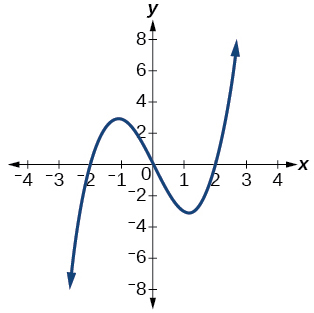Exercise 1.1.41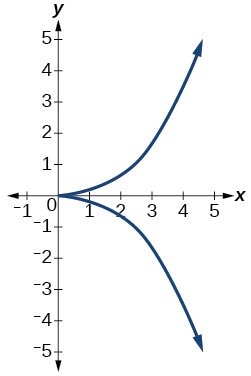Solution

not a function

Exercise 1.1.42Exercise 1.1.43Solution

function

Exercise 1.1.44Exercise 1.1.45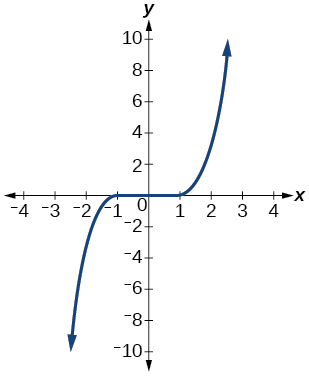Solution

function

Exercise 1.1.46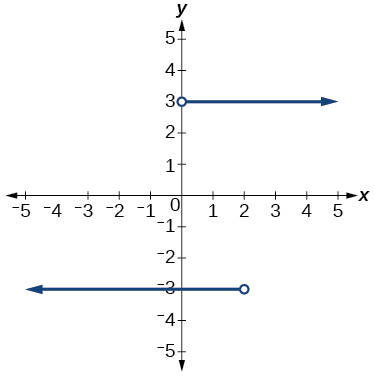Exercise 1.1.47Solution

function

Exercise 1.1.48Exercise 1.1.49Solution

function

Exercise 1.1.50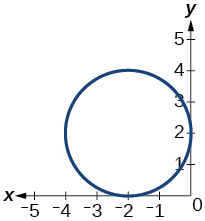Exercise 1.1.51Solution

function

Exercise 1.1.52

Given the following graph,

• Evaluate $$f(−1)$$.
• Solve for $$f(x)=3$$.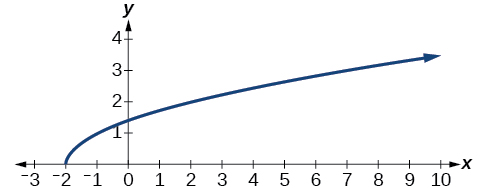Exercise 1.1.53

Given the following graph,

• Evaluate $$f(0)$$.
• Solve for $$f(x)=−3$$.Solution

a.$$f(0)=1$$; b. $$f(x)=−3$$, $$x=−2$$ or $$x=2$$

Exercise 1.1.54

Given the following graph,

• Evaluate $$f(4)$$.
• Solve for $$f(x)=1$$.For the following exercises, determine if the given graph is a one-to-one function.

Exercise 1.1.55Solution

not a function so it is also not a one-to-one function

Exercise 1.1.56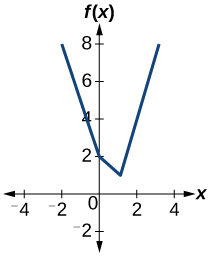Exercise 1.1.57Solution

one-to- one function

Exercise 1.1.58Exercise 1.1.59Solution

function, but not one-to-one

Numeric

For the following exercises, determine whether the relation represents a function.

Exercise 1.1.60

$$\{(−1,−1),(−2,−2),(−3,−3)\}$$

Exercise 1.1.61

$$\{(3,4),(4,5),(5,6)\}$$

Solution

function

Exercise 1.1.62

$$(2,5),(7,11),(15,8),(7,9)\}$$

For the following exercises, determine if the relation represented in table form represents $$y$$ as a function of $$x$$.

Exercise 1.1.63

 $$x$$ 5 10 15 $$y$$ 3 8 14
Solution

function

Exercise 1.1.64

 $$x$$ 5 10 15 $$y$$ 3 8 8

Exercise 1.1.65

 $$x$$ 5 10 10 $$y$$ 3 8 14

Solution

not a function

For the following exercises, use the function f represented in Table 1.1.14.

 $$x$$ $$f(x)$$ 0 74 1 28 2 1 3 53 4 56 5 3 6 36 7 45 8 14 9 47

Table 1.1.14

Exercise 1.1.66

Evaluate $$f(3)$$.

Exercise 1.1.67

Solve $$f(x)=1$$.

Solution

$$f(x)=1$$, $$x=2$$

For the following exercises, evaluate the function $$f$$ at the values $$f(−2)$$, $$f(−1)$$, $$f(0)$$, $$f(1)$$,and $$f(2)$$.

Exercise 1.1.68

$$f(x)=4−2x$$

Exercise 1.1.69

$$f(x)=8−3x$$

Solution

$$f(−2)=14$$;$$f(−1)=11$$;$$f(0)=8$$;$$f(1)=5$$;$$f(2)=2$$

Exercise 1.1.70

$$f(x)=8x^2−7x+3$$

Exercise 1.1.71

$$f(x)=3+\sqrt{x+3}$$

Solution

$$f(−2)=4$$; $$f(−1)=4.414$$; $$f(0)=4.732$$; $$f(1)=4.5$$; $$f(2)=5.236$$

Exercise 1.1.72

$$f(x)=\dfrac{x-2}{x+3}$$

Exercise 1.1.73

$$f(x)=3x$$

Solution

$$f(−2)=19$$; $$f(−1)=13$$; $$f(0)=1$$; $$f(1)=3$$; $$f(2)=9$$

For the following exercises, evaluate the expressions, given functions $$f$$, $$g$$, and $$h$$:

• $$f(x)=3x−2$$
• $$g(x)=5−x^2$$
• $$h(x)=−2x^2+3x−1$$

Exercise 1.1.74

$$3f(1)−4g(−2)$$

Exercise 1.1.75

$$f\Big(\dfrac{7}{3}\Big)−h(−2)$$

Solution

20

Technology

For the following exercises, graph $$y=x^2$$ on the given viewing window. Determine the corresponding range for each viewing window. Show each graph.

Exercise 1.1.76

$$[−0.1, 0.1]$$

Exercise 1.1.77

$$[−10, 10]$$

Solution

$$[0, 100]$$Exercise 1.1.78

$$[−100,100]$$

For the following exercises, graph $$y=x^3$$ on the given viewing window. Determine the corresponding range for each viewing window. Show each graph.

Exercise 1.1.79

$$[−0.1, 0.1]$$

Solution

$$[−0.001, 0.001]$$Exercise 1.1.80

$$[−10, 10]$$

Exercise 1.1.81

$$[−100, 100]$$

Solution

$$[−1,000,000, 1,000,000]$$For the following exercises, graph $$y=\sqrt{x}$$ on the given viewing window. Determine the corresponding range for each viewing window. Show each graph.

Exercise 1.1.82

$$[0, 0.01]$$

Exercise 1.1.83

$$[0, 100]$$

Solution

$$[0, 10]$$Exercise 1.1.84

$$[0, 10,000]$$

For the following exercises, graph $$y=\sqrt{x}$$ on the given viewing window. Determine the corresponding range for each viewing window. Show each graph.

Exercise 1.1.85

$$[−0.001,0.001]$$

Solution

$$[−0.1,0.1]$$Exercise 1.1.86

$$[−1000,1000]$$

Exercise 1.1.87

$$[−1,000,000,1,000,000]$$

Solution

$$[−100, 100]$$Real-World Applications

Exercise 1.1.88

The amount of garbage, $$G$$, produced by a city with population $$p$$ is given by $$G=f(p)$$. $$G$$ is measured in tons per week, and p is measured in thousands of people.

The town of Tola has a population of 40,000 and produces 13 tons of garbage each week. Express this information in terms of the function $$f$$.Tola has a population of 40,000 and produces 13 tons of garbage each week. Express this information in terms of the function $$f$$.
Explain the meaning of the statement $$f(5)=2$$.

Exercise 1.1.89

The number of cubic yards of dirt, $$D$$, needed to cover a garden with area a square feet is given by $$D=g(a)$$.

a. A garden with area 5000 $$ft^2$$ requires 50 yd3 of dirt. Express this information in terms of the function $$g$$.
b. Explain the meaning of the statement $$g(100)=1$$.

Solution

a. $$g(5000)=50$$; b. The number of cubic yards of dirt required for a garden of 100 square feet is 1.

Exercise 1.1.90

Let $$f(t)$$ be the number of ducks in a lake $$t$$ years after 1990. Explain the meaning of each statement:

a. f(5)=30
b. f(10)=40

Exercise 1.1.91

Let $$h(t)$$ be the height above ground, in feet, of a rocket $$t$$ seconds after launching. Explain the meaning of each statement:

a. $$h(1)=200$$
b. $$h(2)=350$$

Solution

a. The height of a rocket above ground after 1 second is 200 ft. b. the height of a rocket above ground after 2 seconds is 350 ft.

Exercise 1.1.92

Show that the function $$f(x)=3(x−5)2+7$$ is not one-to-one.